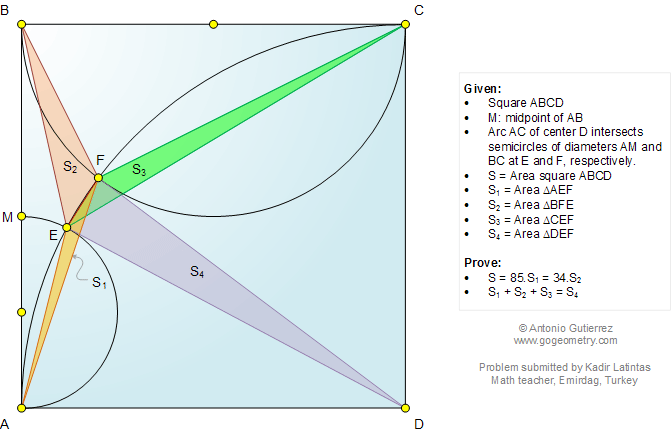# Online Geometry Problem 1051: Triangle, Square, Semicircle, Arc, Area. Level: High School, SAT Prep, College, Mathematics Education

< PREVIOUS PROBLEM  |  NEXT PROBLEM >

 The figure below shows a square ABCD with M midpoint of AB. Arc AC of center D intersects the semicircles of diameters AM and BC at E and F, respectively. If S is the area of region ABCD, and S1, S2, S3, and S4 are the areas of regions AEF, BFE, CEF, and DEF, respectively, prove that (1) S = 85S1 = 34S2; (2) S1 + S2 + S3 = S4. Problem submitted by Kadir Latintas, Math teacher in Emirdag, Turkey.Home | Search | Geometry | Problems | All Problems | Open Problems | Visual Index | 10 Problems | 1051-1060 | Triangle | Square | Circle | Areas | Triangle area | Midpoint | Email | Solution / comment | By Antonio Gutierrez Last updated: Oct 30, 2014